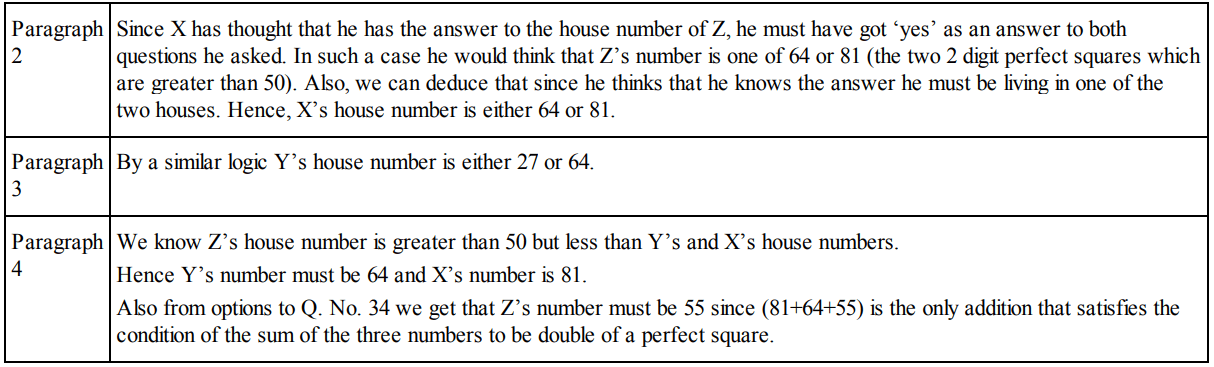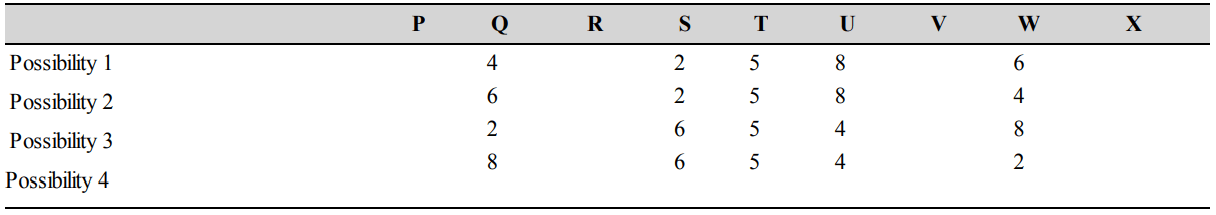## Quantitative Reasoning Questions and Answers Part-4

Data for Questions 1 to 4 : Three classmates—X, Y and Z live on the AN Jha Marg, yet they do not know the house number of each other. The houses are numbered from 1 to 99. Since Z is a regular student and attends every class sincerely, his notes are very good and updated. X and Y are not so regular, therefore they desire to meet Z at his house individually.
One day X asks Z, “The number of your house in which you reside is a perfect square or not?” Z replies. Then X asks, “Is it greater than 50?” He again replies. X thinks that he has got the address and decides to visit Z. When X reaches at the address he realises that he is wrong. He then thinks over it again and is not surprised as Z answered only the second question honestly.
Y not aware of X’s conversation, asks Z two questions of his own. Y asks “Is your house number a perfect cube?” Z replies. Then Y asks “Is it greater than 25?”
He answers again. Y thinks that he has got the address but upon reaching there he finds the address incorrect and realises that Z answered only the second question honestly.
If Z’s house number is less than the house number of X and Y and the sum of all three of their house numbers is twice the perfect square of some number then answer the following question:

1. What is X’s house number?
a) 64
b) 81
c) 49
d) Cannot be determined

Explanation:The answer is 81. Option (b) is correct

2. What is Y’s house number?
a) 64
b) 81
c) 36
d) Cannot be determined

Explanation: The answer is 64. Option (a) is correct (Refer explanation of Question 1)

3. What is Z’s house number?
a) 55
b) 65
c) 25
d) 85

Explanation: The answer is 55. Option (a) is correct (Refer explanation of Question 1)

4. What is the sum of house numbers of all the three X, Y and Z?
a) 100
b) 200
c) 128
d) Cannot be determined

Explanation: The answer is 200. Option (b) is correct (Refer explanation of Question 1)

Data for Questions 5 to 10 : It is very easy to remember the ID number of my ATM card which is a nine digit number and every digit is distinct. If I tell you some clues then you will also be able to remember my ATM card ID number. Let us say the number is PQRSTUVWX and the digit corresponding to it are 1 to 9 though not respectively. The ID is divisible by 9.
If you delete the digit at its units place, the remaining 8-digit number of my ID is divisible by 8. If you again delete the last digit of the 8-digit number the remaining 7-digit number is divisible by 7 and the process goes on.

5. What is the sum of the digits of the ID number of my ATM card?
a) 55
b) 45
c) 90
d) Cannot be determined

Explanation: The alphabets PQRSTUVWX represent the nine digit number with the given property.
Deduction1: Since all numbers having even digits have to be divisible by even numbers (i.e., PQ is divisible by 2, PQRS is divisible by 4, PQRSTU is divisible by 6 and PQRSTUVW is divisible by 8), Q, S, U & W must be the 4 even numbers viz. 2, 4, 6 and 8 in some random order.
Consequently P, R, T, V & X (the odd placed digits) must be sharing the odd digits 1,3, 5,7 and 9 in some order.
Deduction 2: Since PQRST is divisible by 5, T must be equal to 5. Thus, the number must be PQRS5UVWX.
Deduction 3: PQR is divisible by 3 and PQRSTU is divisible by 6, hence STU must be divisible by 3. i.e., S + T + U = S + 5 + U must be divisible by 3. Also, S and U are even number. Through trial and error you should realise that there are only two ways this could happen:
(a) lf STU represents 258
(b) lf STU represents 654
Note: S can only be 2 or 6 since for PQRS to be divisible by 4, RS should be divisible by 4 and R being an odd number S can only be 2 or 6.
Deduction 4: The following 4 options of filling in the other two even numbers (4 and 6 in case STU is 258 or 2 and 8 in case STU is 654) emerge:Sum of digits—1+2+3+4+5+6+7+8+9=45
Option (b) is correct

6. What is the digit sum of the ID number of my ATM?
a) 9
b) 8
c) 3
d) Cannot be determined

Explanation: Digit sum = 4 + 5 = 9. Option (a) is correct (Refer explanation of Question 5)

7. What is the number represented by the letter R?
a) 9
b) 8
c) 1
d) Cannot be determined

Explanation: R = 1. Option (c) is correct (Refer explanation of Question 5)

8. The number 2 represents which letter?
a) V
b) W
c) X
d) Cannot be determined

Explanation: 2 = W. Option (b) is correct (Refer explanation of Question 5)

9. What are the first 5-digits of the ID number of my ATM card?
a) 38165
b) 61853
c) 65472
d) 56427